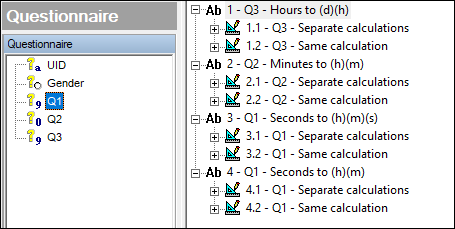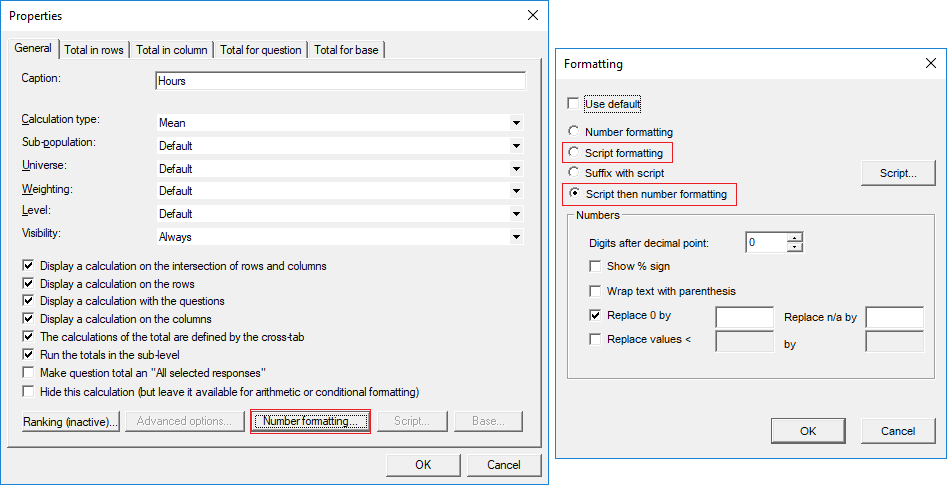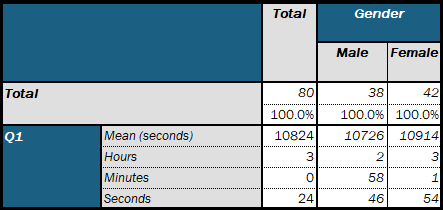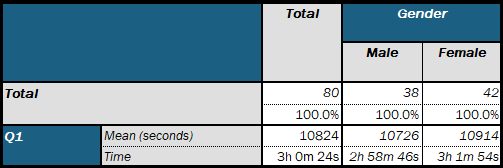Scroll

# Converting Time Values

Follow
 Summary This article describes how to convert the numeric calculations for time values in hours, minutes or seconds to days+hours, hours+mins, hours+mins+seconds respectively. to Askia Analyse Written for Data Processors, Analysts. Keywords Days, Hours, Minutes, Seconds, Convert, Time, Average, Mean, Numeric, Value, Script formatting, Script then number formatting.

We will focus on the mean score calculation but it can be applied to other numeric calculations such as standard deviation or standard error etc.

In the portfolio we have 4 chapters where we carry out a different conversion.In each section we have:

1. One tab definition where we return a number into multiple time calculations (Mean + Script then number formatting)
2. One tab definition where we return text into a single time calculation e.g. "1h 50m" (Mean + Script formatting)The results will look like this:

1.2.I've listed out all the format scripts required below for easy reference. The keyword {1} in script formatting refers to 'this current calculation' i.e. the mean value in its original units.

Q3 - Hours to (d)(h):

Separate calculations (script then number formatting):

Days:

`int({1}/24)`

Hours:

`{1}-((int({1}/24)*24))`

Same calculation (script formatting):

Time:

`int({1}/24) + "d " + int({1}-((int({1}/24)*24))) + "h"`

Q2 - Minutes to (h)(m):

Separate calculations (script then number formatting):

Hours:

`int({1}/60)`

Minutes:

`{1}-((int({1}/60)*60))`

Same calculation (script formatting):

Time:

`int({1}/60) + "d " + int({1}-((int({1}/60)*60))) + "h"`

Q1 - Seconds to (h)(m)(s):

Separate calculations (script then number formatting):

Hours:

`int({1}/(60*60))`

Minutes:

`int(({1}-((int({1}/(60*60))*60*60)))/60)`

Seconds:

`{1}-((int({1}/60)*60))`

Same calculation (script formatting):

Time:

`int({1}/(60*60)) + "h " + int(({1}-((int({1}/(60*60))*60*60)))/60) + "m " + ({1}-((int({1}/60)*60))).Round() + "s"`

Q1 - Seconds to (h)(m):

Separate calculations (script then number formatting):

Hours:

`int({1}/(60*60))`

Minutes:

`(({1}-((int({1}/(60*60))*60*60)))/60)`

Same calculation (script formatting):

Time:

`int({1}/(60*60)) + "h " + (({1}-((int({1}/(60*60))*60*60)))/60).Round() + "m"`

Lastly, if you would like your single digit numbers to be preceded by a zero then you could get around it by writing a conditional script formatting e.g.

```If int({1}- ((int({1}/60)*60))) < 10 Then
Return int({1}/60) + ".0" + int({1}- ((int({1}/60)*60))) + " pm"
Else
Return int({1}/60) + "." + int({1}- ((int({1}/60)*60))) + " pm"
EndIf
```